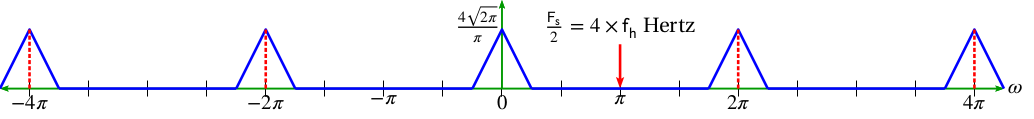# Oversampling

August 5, 2019

Rather than sampling at the Nyquist frequency as in Example 5 or sampling at twice the Nyquist frequency as in Example 4, there can be sampling at 4 times or even 256 times the Nyquist rate.

Sampling higher than the Nyquist rate is called over-sampling. As the sample rate increases, the spectral images spread farther as well as “taller” in the DTFT frequency domain. The significance of over-sampling is discussed later in this course in the context of quantization noise and delta-sigma modulation.

### Frequency Content of Over-sampled Waveform (Ex. 6)

Figure 2.8 illustrates the spectral content of the waveform x(t) sampled at 𝖥s = 8  × 𝖿h rather than 4  × 𝖿h.Figure 2.8. The frequency content of an over-sampled waveform.

There is still a repeat every 𝜔 = 2𝜋 as in Example 4, but the images also become more narrow and thus more “spread out” in the DTFT frequency domain.